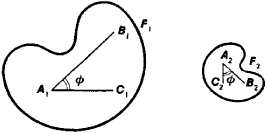# Similarity

Also found in: Dictionary, Thesaurus, Wikipedia.
The following article is from The Great Soviet Encyclopedia (1979). It might be outdated or ideologically biased.

## Similarity

a concept in geometry. Geometric figures are said to be similar if they are identical in shape, regardless of whether they are identical in size. The figures F1 and F2 are similar if between their points a one-to-one correspondence can be established such that the ratio of the distances between any two pairs of corresponding points of F1 and F2 a constant k. This constant is called the similarity factor. The angles between corresponding lines of similar figures are equal. Thus, in Figure 1 ∠B1A11C1 = ∠B2A2C2 = ϕ. The ratio of the areas of bounded similar figures is equal to the square of the similarity factor, and the ratio of the volumes of similar solids is equal to the cube of the similarity factor.Figure 1

A geometric transformation of the plane (or of space) such that all figures in the plane are transformed into similar figures with the same ratio of similitude is called a similarity transformation, which is a special case of an affine transformation. The set of all similarity transformations of the plane or of space forms a group. Any similarity transformation can be represented as the successive application of a homothetic transformation and a proper or improper motion.

The concept of similarity and similarity transformations are used in such engineering applications of geometry as modeling and drawing. For example, the operation of the pantograph is based on the concept of similarity.

References in periodicals archive ?
The maximum frequency of the spatial similarity values happens at the range of 0.05, 0.1 and then it decreases until the range of 0.45, 0.5.
Results on Correlation between Spatial Similarity and Temporal Similarity.
Distance, entropy and similarity measures are the central arenas that are investigated by various researchers under intuitionistic and interval-valued fuzzy environment (IFE and IvFE).
Cosine similarity is a method used to calculate a similarity or similarity between two objects.
Ye  proposed an MADM method with completely unknown weights based on similarity measures under SVNS environment.
The similarity index of the research paper was 59%, while 26% was plagiarised from a single source.
Proposing a mechanism to explain the unusually high level of similarity, researchers show that it is associated with risk of cancer in adulthood, according to the study.
In visual analytics, similarity mining is a machine learning method based on the analysis of similarities of the distance measures and has been recently adopted to detect malware.
On the other hand, the cosine similarity measure based on Bhattacharyya distance was first proposed in Bhattacharyya .
It has been previously shown that group affiliation influences perceived similarity and results in higher cooperation rates (e.g., Fischer 2009.
The application of fuzzy numbers in resolving problems requires the application of different similarity measures, calculating the degree of similarity between two fuzzy number; similarity measures appropriate for solving one type of problem often are not appropriate for solving the other problem or type of problems.

Site: Follow: Share:
Open / Close